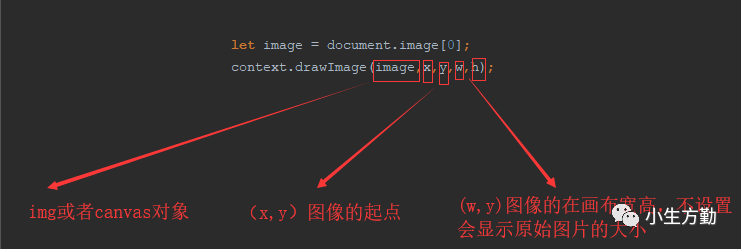# Canvas 动画，下雪了

## 入题

UI 的需求是雪花下落的方向有点倾斜角度，每片雪花的下落速度不一样但要保持在一个范围内。

### drawImagedrawImage 可传入 9 个参数，上图中的 5 个参数是比较常用的，另外几个参数是拿来剪切图片的。

### requestAnimationFrame

requestAnimationFrame 相对于 setinterval 处理动画有以下几个优势：

1. 经过浏览器优化，动画更流畅

2. 窗口没激活时，动画将停止，节省计算资源

3. 更省电，尤其是对移动终端

window.requestAnimationFrame = (function(){
return  window.requestAnimationFrame       ||
window.webkitRequestAnimationFrame ||
window.mozRequestAnimationFrame    ||
window.oRequestAnimationFrame      ||
window.msRequestAnimationFrame     ||
function (callback) {
window.setTimeout(callback, 1000 / 60);
};
})();


## 第一次尝试

function preloadImg(srcArr){
if(srcArr instanceof Array){
for(let i = 0; i < srcArr.length; i++){
let oImg = new Image();
oImg.src = srcArr[i];
}
}
}


## 第二次尝试前的准备

### 1. 使用多层画布绘制复杂场景

<canvas style="position: absolute; z-index: 0"></canvas>
<canvas style="position: absolute; z-index: 1"></canvas>
// js 代码


### 4. 使用离屏绘制进行预渲染

1. 若数据源（图片、canvas）和 canvas 画板的尺寸相仿，那么性能会比较好；

2. 若数据源只是大图上的一部分，那么性能就会比较差；因为每一次绘制还包含了裁剪工作。

drawImage 方法的第一个参数不仅可以接收 Image 对象，也可以接收另一个 Canvas 对象。而且，使用 Canvas 对象绘制的开销与使用 Image 对象的开销几乎完全一致。

let cacheCanvas = document.createElement("canvas");
let cacheCtx = this.cacheCanvas.getContext("2d");
cacheCtx.save();
cacheCtx.lineWidth = 1;
for(let i = 1;i < 40; i++){
cacheCtx.beginPath();
cacheCtx.strokeStyle = this.color[i];
cacheCtx.arc(this.r , this.r , i , 0 , 2*Math.PI);
cacheCtx.stroke();
}
this.cacheCtx.restore();
// 在绘制每一帧的时候，绘制这个图形
context.drawImage(cacheCtx, x, y);


cacheCtx 的宽高尽量设置成实际使用的宽高，否则过多空白区域也会造成性能的损耗。

### 5. 尽量少调用 canvasAPI ，尽可能集中绘制

for (var i = 0; i < points.length - 1; i++) {
var p1 = points[i];
var p2 = points[i + 1];
context.beginPath();
context.moveTo(p1.x, p1.y);
context.lineTo(p2.x, p2.y);
context.stroke();
}


context.beginPath();
for (var i = 0; i < points.length - 1; i++) {
var p1 = points[i];
var p2 = points[i + 1];
context.moveTo(p1.x, p1.y);
context.lineTo(p2.x, p2.y);
}
context.stroke();


tips: 写粒子效果时，可以使用方形替代圆形，因为粒子小，所以方和圆看上去差不多。有人问为什么？很容易理解，画一个圆需要三个步骤：先 beginPath，然后用 arc 画弧，再用 fill。而画方只需要一个 fillRect。当粒子对象达一定数量时性能差距就会显示出来了。

### 6. 像素级别操作尽量避免浮点运算

function getInt(num){
var rounded;
rounded = (0.5 + num) | 0;
return rounded;
}


## 第二次尝试

1. 使用离屏绘制进行预渲染

2. 减少部分 API 的使用

3. 浮点数取整

4. 缓存变量

5. 使用 for 循环，替代 forEach

6. 将整体代码使用原型链方式改写了一遍

200 片雪花的时候 FPS 基本稳定在 60，而且抖动的情况也没了；

### 主要代码

let snowBox = function () {
let canvasEl = document.getElementById("snowFall");
let ctx = canvasEl.getContext( 2d );
canvasEl.width = window.innerWidth;
canvasEl.height = window.innerHeight;
let lineList = []; // 雪的容器
let snow = function () {
let _this = this;
_this.cacheCanvas = document.createElement("canvas");
_this.cacheCtx = _this.cacheCanvas.getContext("2d");
_this.cacheCanvas.width = 10;
_this.cacheCanvas.height = 10;
_this.speed = [1, 1.5, 2][Math.floor(Math.random()*3)];                // 雪花下落的三种速度，便于取整
_this.posx = Math.round(Math.random() * canvasEl.width);               // 雪花x坐标
_this.posy = Math.round(Math.random() * canvasEl.height);              // 雪花y坐标
_this.img = ./img/snow_(\${Math.ceil(Math.random() * 9)}).png;        // img
_this.w = _this.getInt(5 + Math.random() * 6);
_this.h = _this.getInt(5 + Math.random() * 6);
_this.cacheSnow();
};
snow.prototype = {
cacheSnow: function () {
let _this = this;
// _this.cacheCtx.save();
let img = new Image();   // 创建img元素
img.src = _this.img;
_this.cacheCtx.drawImage(img, 0, 0, _this.w, _this.h);
// _this.cacheCtx.restore();
},
fall: function () {
let _this = this;
if (_this.posy > canvasEl.height + 5) {
_this.posy = _this.getInt(0 - _this.h);
_this.posx = _this.getInt(canvasEl.width * Math.random());
}
if (_this.posx > canvasEl.width + 5) {
_this.posx = _this.getInt(0 - _this.w);
_this.posy = _this.getInt(canvasEl.height * Math.random());
}
// 如果雪花在可视区域
if (_this.posy <= canvasEl.height || _this.posx <= canvasEl.width) {
_this.posy = _this.posy + _this.speed;
_this.posx = _this.posx + _this.speed * .5;
}
_this.paint();
},
paint: function () {
ctx.drawImage(this.cacheCanvas, this.posx, this.posy)
},
getInt: function(num){
let rounded;
rounded = (0.5 + num) | 0;
return rounded;
}
};
let control;
control = {
start: function (num) {
for (let i = 0; i < num; i++) {
let s = new snow();
lineList.push(s);
}
(function loop() {
ctx.clearRect(0, 0, canvasEl.width, canvasEl.height);
for (let i = 0; i < num; i++) {
lineList[i].fall();
}
requestAnimationFrame(loop)
})();
}
};
return control;
}();
snowBox.start(2000)
};


## 后话

1. 减少 API 的使用

2. 使用缓存（重点）

3. 合并频繁使用的 API

4. 避免使用高耗能的 API

5. 用 webWorker 来处理一些比较耗时的计算

6. ……06-22
07-141622
04-25
03-20
12-18622
05-25623
10-19140
03-1387
06-077
08-28点击重新获取扫码支付余额充值